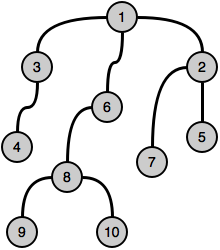Recent Tutorials and Articles
HackerRank Solution: Even Tree
Published on: 25th May 2018

This tutorial provides Java solution to "Even Tree" challenge of HackerRank.

# Hackerrank Challenge Details

### Problem Statement:

You are given a tree (a simple connected graph with no cycles). The tree has N nodes numbered from 1 to N.

Find the maximum number of edges you can remove from the tree to get a forest such that each connected component of the forest contains an even number of vertices.

### Input Format:

The first line of input contains two integers N and M. N is the number of vertices, and M is the number of edges.
The next M lines contain two integers ui and vi which specifies an edge of the tree.

### Output Format:

Print the number of removed edge..

### Constraints:

• 2 < N < 100

### Sample Input:

``````10 9
2 1
3 1
4 3
5 2
6 1
7 2
8 6
9 8
10 8``````

### Sample Output:

``2``

### Explanation:

On removing edges (1, 3) and (1, 6), we can get the desired result.

Original tree:Decomposed tree:# Solution Details

## Java Implementation:

``````package com.saintech.allprogtutorials.hackerrank.algos;

import java.util.ArrayList;
import java.util.List;
import java.util.Scanner;

/**
* @author Sain Technology Solutions
*
* Solution to Problem - https://www.hackerrank.com/challenges/even-tree
*
*/
public class EvenTree {

/**
* Represents Node of the graph and contains the nodes that are attached to it. We may
* also have ids but emitted as array index is being used as node id.
*/
private static class Node<T> {
private final List<Node<T>> childNodes = new ArrayList<>();

}
}

/**
* Returns an array of integer containing below information about tree represented by input node:
* 	- No of vertices
* 	- Edges removed to decompose tree into trees of even vertices
*/
private static int[] decomposeTree(Node<Integer> node) {

int count = 0, edgesToRemove = 0;
for(Node<Integer> childNode : node.childNodes) {
// For each child node
// calls current method recursively
// Accumulates edgesToRemove in a local variable
// Checks if returned count is even - if yes, increments edgesToRemove by 1
// otherwise adds returned count to this method's count
if(tmpMetadata % 2 == 0) {
edgesToRemove++;
} else {
}
}
// Increments count since we are done with current node processing
count++;
//returns count for input node along with edges that can be removed to decompose the tree
return new int[]{count, edgesToRemove};
}

public static void main(String[] args) {
final Scanner in = new Scanner(System.in);
final int N = in.nextInt();
final int M = in.nextInt();

// Keeps all the nodes of tree in an array
final Node<Integer>[] treeNodes = (Node<Integer>[]) new Node[N];
for(int i = 0; i < M; i++) {
// Gets first node of vertex
final int node1 = in.nextInt() - 1;
// Gets second node of vertex
final int node2 = in.nextInt() - 1;

// If nodes exist in array, reuses those else creates a new node and adds back to array
treeNodes[node1] = (treeNodes[node1] == null ? new Node<Integer>() : treeNodes[node1]);
treeNodes[node2] = (treeNodes[node2] == null ? new Node<Integer>() : treeNodes[node2]);

//In order to create edge, we need to link nodes. Since this is a tree, it will be a directed edge and
// for consistency, we will add bigger node to smaller nodes
if(node1 < node2) {
} else {
}
}

// Since first element of array represents root of tree, we pass it to recursive method decompose tree

// Print no of edges to be removed returned by above method invocation